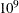### ODS Tables

PROC OPTLP creates three Output Delivery System (ODS) tables by default. The first table, ProblemSummary, is a summary of the input LP problem. The second table, SolutionSummary, is a brief summary of the solution status. The third table, PerformanceInfo, is a summary of performance options. You can use ODS table names to select tables and create output data sets. For more information about ODS, see SAS Output Delivery System: Procedures Guide.

If you specify a value of 2 for the PRINTLEVEL= option, then the ProblemStatistics table is produced. This table contains information about the problem data. For more information, see the section Problem Statistics. If you specify PRINTLEVEL=2 and ALGORITHM=CON, the ConcurrentSummary table is produced. This table contains solution status information for all algorithms that are run concurrently. For more information, see the section Concurrent LP.

If you specify the DETAILS option in the PERFORMANCE statement, then the Timing table is produced.

Table 10.5 lists all the ODS tables that can be produced by the OPTLP procedure, along with the statement and option specifications required to produce each table.

Table 10.5: ODS Tables Produced by PROC OPTLP

ODS Table Name

Description

Statement

Option

ProblemSummary

Summary of the input LP problem

PROC OPTLP

PRINTLEVEL=1 (default)

SolutionSummary

Summary of the solution status

PROC OPTLP

PRINTLEVEL=1 (default)

ProblemStatistics

Description of input problem data

PROC OPTLP

PRINTLEVEL=2

ConcurrentSummary

Summary of the solution status for all algorithms run concurrently

PROC OPTLP

PRINTLEVEL=2, ALGORITHM=CON

PerformanceInfo

List of performance options and their values

PROC OPTLP

PRINTLEVEL=1 (default)

Timing

Detailed solution timing

PERFORMANCE

DETAILS

A typical output of PROC OPTLP is shown in Figure 10.2.

Figure 10.2: Typical OPTLP Output

The OPTLP Procedure

Problem Summary
Objective Sense Minimization
Objective Function .Z....
RHS ZZZZ0001

Number of Variables 97
Bounded Above 0
Bounded Below 97
Bounded Above and Below 0
Free 0
Fixed 0

Number of Constraints 56
LE (<=) 40
EQ (=) 15
GE (>=) 1
Range 0

Constraint Coefficients 383

Performance Information
Execution Mode Single-Machine
Number of Threads 4

Solution Summary
Solver LP
Algorithm Dual Simplex
Objective Function .Z....
Solution Status Optimal
Objective Value 225494.96316

Primal Infeasibility 2.273737E-13
Dual Infeasibility 1.847411E-13
Bound Infeasibility 0

Iterations 77
Presolve Time 0.00
Solution Time 0.06

You can create output data sets from these tables by using the ODS OUTPUT statement. This can be useful, for example, when you want to create a report to summarize multiple PROC OPTLP runs. The output data sets corresponding to the preceding output are shown in Figure 10.3, where you can also find (at the row following the heading of each data set in display) the variable names that are used in the table definition (template) of each table.

Figure 10.3: ODS Output Data Sets

 Problem Summary

Obs Label1 cValue1 nValue1
1 Problem Name ADLITTLE .
2 Objective Sense Minimization .
3 Objective Function .Z.... .
4 RHS ZZZZ0001 .
5     .
6 Number of Variables 97 97.000000
7 Bounded Above 0 0
8 Bounded Below 97 97.000000
9 Bounded Above and Below 0 0
10 Free 0 0
11 Fixed 0 0
12     .
13 Number of Constraints 56 56.000000
14 LE (<=) 40 40.000000
15 EQ (=) 15 15.000000
16 GE (>=) 1 1.000000
17 Range 0 0
18     .
19 Constraint Coefficients 383 383.000000

 Solution Summary

Obs Label1 cValue1 nValue1
1 Solver LP .
2 Algorithm Dual Simplex .
3 Objective Function .Z.... .
4 Solution Status Optimal .
5 Objective Value 225494.96316 225495
6     .
7 Primal Infeasibility 2.273737E-13 2.273737E-13
8 Dual Infeasibility 1.847411E-13 1.847411E-13
9 Bound Infeasibility 0 0
10     .
11 Iterations 77 77.000000
12 Presolve Time 0.00 0
13 Solution Time 0.06 0.062401

#### Problem Statistics

Optimizers can encounter difficulty when solving poorly formulated models. Information about data magnitude provides a simple gauge to determine how well a model is formulated. For example, a model whose constraint matrix contains one very large entry (on the order of) can cause difficulty when the remaining entries are single-digit numbers. The PRINTLEVEL=2 option in the OPTLP procedure causes the ODS table ProblemStatistics to be generated. This table provides basic data magnitude information that enables you to improve the formulation of your models.

The example output in Figure 10.4 demonstrates the contents of the ODS table ProblemStatistics.

Figure 10.4: ODS Table ProblemStatistics

The OPTLP Procedure

Problem Statistics
Number of Constraint Matrix Nonzeros 8
Maximum Constraint Matrix Coefficient 3
Minimum Constraint Matrix Coefficient 1
Average Constraint Matrix Coefficient 1.875

Number of Objective Nonzeros 3
Maximum Objective Coefficient 4
Minimum Objective Coefficient 2
Average Objective Coefficient 3

Number of RHS Nonzeros 3
Maximum RHS 7
Minimum RHS 4
Average RHS 5.3333333333

Maximum Number of Nonzeros per Column 3
Minimum Number of Nonzeros per Column 2
Average Number of Nonzeros per Column 2

Maximum Number of Nonzeros per Row 3
Minimum Number of Nonzeros per Row 2
Average Number of Nonzeros per Row 2